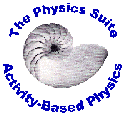PI Problems for the Physics Suite Edward F. Redish

Peer Instruction Problems:
General and Mathematics

 Problem Name Comments Source G1. Winter days This is a good RAD warm up. The answer is ambiguous and any one of the three answers can be acceptible depending on how you interpret the wording. EFR G2. Australia Another good ambiguous RAD warm up. EFR G3. Estimation A nice problem to introduce the students to estimation. If they have identified the first joint of their thumb as ~1 inch long (2.5 cm) they can do this as their seats. Note that a dimension conversion equation is somewhat peculiar from a mathematical point of view where dimensions are not usually considered, but not from the physics perspective where variables represent physical quantities that can be measured in different ways, not just numbers. Based on a problem from SCALE-UP, courtesy Bob Beichner G4. Dimensional Analysis An example that uses dimensional analysis to check the validity of equations. Students in introductory physics often do not see the value of checking dimensions. EFR G5. Scaling Measurements 1 A problem that helps them focus on the critical point of dimensional analysis: it tells how quantitites change when the scales change. This problem focuses on how quantities change when the measurement scale changes. EFR G6. Scaling Measurements 2 Another problem that helps them focus on the critical point of dimensional analysis: it tells how quantitites change when the scales change. This problem focuses on how quantities change when the size of the object changes. EFR G7. Vector Aerobics A vector addition and subtraction practice. I hold the 2-D vector analysis until we have a physical need for them through analysis of forces and motions in 2-D. EFRWork supported in part by a grant from the US National Science Foundation.

Maintained by Edward F. Redish
Comments and questions may be directed to redish@umd.edu